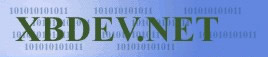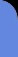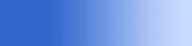Friday June 5, 2020
 home | about | contact | DonationsTutorials - Tips - Secrets - for C and C++.. It does all the hard work for us..

by bkenwright@xbdev.net

A simple thing to overload is the "Assignment Operator" (=) ...which saves a lot of time when you want to copy the data from one class to another.

Lets take an example of the "default" assigment operator.... as if you don't tell C++ that you've done one...it gets clever and does its own!  But its dangerous!  Especially with pointers and allocated memory :-(

As in the below example we have created a simple class....and we create two instances of it, and of course use the "="...equal thingy to set 'a' equal to 'b'.... it does this with each piece of data in the class:

a::data = b::data;

...repeat for all the data values...

This is okay for simple classes... but classes that use pointers, we want to use our own one.....

 Code: #include   // Assignment operator ... e.g. the equals thing.. "="   // Demo Class class CSimple { public:       int data; };   void main() {       cout << "Enter Our Program\n";         CSimple a;       CSimple b;       a = b;         cout << "Leave Our Program - Bye-Bye\n"; }

Note:

Optimisation is a big thing with coding... and will always pop up from time to time... now the default operators all pass by value...which means the constructor/descructor always get called when you use the "=" operator for example...also...when a value is returned...its returned by value....which again means another instance is created/destroyed.  Of course it nothing much to probably worry about... but push it to the back of your mind...and keep it there...as its always sweet to know :)

Self Note: Copy Constructor => CSimple a(b);

Lets open up a can of creativity, and try out something...lets implement what the classes look like with some operator overloading and a copy constructor...a default home made constructor etc..

 Code: // Demo Class class CSimple { public:       int data;         CSimple() { cout << "Default Empty Construtor\n"; };       CSimple(int d) { cout << "Data Constructor\n"; };       CSimple(CSimple& s) { cout << "Copy Constructor\n"; };         void operator = (CSimple s) // overloaded = operator       {             data = s.data;             cout << "Assigment Operator\n";       } };   void main() {       cout << "Enter Our Program\n";         CSimple a, b, c;       a.data = 4;       b = a;       // a = b = c; // causes a compile error as we havn't returned a value from                     // our operator =         cout << " a data: " << a.data << "\n";       cout << " b data: " << b.data << "\n";           cout << "Leave Our Program - Bye-Bye\n"; }

 Output: Enter Our Program Default Empty Construtor Default Empty Construtor Default Empty Construtor Copy Constructor Assigment Operator a data: 4 b data: 4242008 Leave Our Program - Bye-Bye

Ackk....look at all that... calling constructors... even a copy constructor is getting called! ... Hmmm... this is why I mentioned efficienty before...as passing by reference or pointer can save a lot of those constructor/destructor calls.

Also take a look at the line "a = b = c" which of course I've commented out...because if I don't return a value from our overloaded = operator...we can't truncate them...Understanding how the data and operator works is important....so I did a simple sketch above, which I hope helps in your understanding....of course if you truncate your assignment operators...it works from right to left...as I can show with this simple example below:

 Code: // Demo Class class CSimple { public:       int data;         CSimple() { cout << "Default Empty Construtor\n"; };       CSimple(int d) { cout << "Data Constructor\n"; };       CSimple(CSimple& s) { cout << "Copy Constructor\n"; };         CSimple operator = (CSimple s) // overloaded = operator       {             cout << "Data passed to = operator: " << s.data << "\n";               data = s.data + 1; // so we know where the data is at any one time             cout << "Assigment Operator\n";                         return data;       } };   void main() {       cout << "Enter Our Program\n";         CSimple a, b, c;       c.data = 4;       a = b = c;         cout << " a data: " << a.data << "\n";       cout << " b data: " << b.data << "\n";           cout << "Leave Our Program - Bye-Bye\n"; }

 Output: Enter Our Program Default Empty Construtor Default Empty Construtor Default Empty Construtor Copy Constructor Data passed to = operator: 4242056Assigment Operator Data Constructor Data passed to = operator: 4242056 Assigment Operator Data Constructor a data: 4242057 b data: 4242057 Leave Our Program - Bye-Bye

Damm..it didn't work!  Why?  Well If you look at the output, you'll notice that each time we pass data using the = operator...the Data Constructor is called... but if you look at the code in the "Data Constructor" "CSimple(int d)" you'll see its empty... so lets implement it...and see what we get... so we add this code:

CSimple(int d)                 // Data Constructor

{

cout << "Data Constructor\n";

data = d;

};

 Output: Enter Our Program Default Empty Construtor Default Empty Construtor Default Empty Construtor Copy Constructor Data passed to = operator: 4242056Assigment Operator Data Constructor Data passed to = operator: 4242057 Assigment Operator Data Constructor a data: 4242058 b data: 4242057 Leave Our Program - Bye-Bye

But its wrong again :(  Why oh why oh why your saying... but what is the first thing that gets called before we go into our first = operator... its the "Copy Constructor"...which of course I didn't implement again ... I guess we'll have to do it now... so lets add that code in here:

CSimple(CSimple& s)            // Copy Constructor

{

cout << "Copy Constructor\n";

data = s.data;

};

Compiling again....and running it again and what do we get?

 Output: Enter Our Program Default Empty Constructor Default Empty Constructor Default Empty Constructor Copy Constructor Data passed to = operator: 4 Assignment Operator Data Constructor Data passed to = operator: 5 Assignment Operator Data Constructor a data: 6 b data: 5 Leave Our Program - Bye-Bye

Wahoooo..its what we want....I new we'd get there in time... but why does it all happy....and isn't there ways of improving it so the copy constructor doesn't get called or those other data constructors?

Yup of course there is....and its all in the aid of optimisation.... you hear that word a lot from me.... as remember I mentioned before....references and pointers only pass the address...so we don't need to create a copy of it and pass it :)

Lets get out the knife, and dissect this puppy!.. see why its being bad...

 We create two instances of our class:

CSimple a, b;

Which of course will call our default constructor as where not passing anything...simple!

 Now we do this:

a.data = 4;

Just setting the data in the class...which we could have done in the constructor...but we'll get to that later.

 Use our = operator

b = a;

This is a bit tricky to understand, but where passing a copy to the = operator....not a reference or a pointer...but a copy...which means the copy constructor gets called!  As in C++...when ever you need a copy of a class....for example:

CSimple b;

CSimple a(b); // a is a copy of b

void func( CSimple s ) // copy is passed to the function, e.g. if we call it like func(b)....it would be like doing CSimple s(b)

Hmmm.....its a little try to why etc at first...but if you pass by value to a function, where a copy is needed...then the copy constructor is called.

But how about this....what if we pass by 'Reference' to our = operator!.... which means it will get a pointer to our data...and won't need to call the copy constructor...lets check with an example:

//** -- Note passing by reference...this is the change -- that & thing has been added*

CSimple operator = (CSimple& s) // overloaded = operator

{

cout << "Data passed to = operator: " << s.data << "\n";

data = s.data + 1; // so we know where the data is at any one time

cout << "Assigment Operator\n";

return data;

}

 Output: Enter Our Program Default Empty Construtor Default Empty Construtor Default Empty Construtor Data passed to = operator: 4 Assigment Operator Data Constructor Data passed to = operator: 5 Assigment Operator Data Constructor a data: 6 b data: 5 Leave Our Program - Bye-Bye

Fantastic...we don't need that copy constructor now!....the plan is finally coming together.

A final tweek, is the returning of a value...since the return value is being used in the next = operator...why can't we just return a reference?  Well we can...but things to look out for when returning pointers or even references...is to make sure that the pointer or reference isn't in function scope...or won't be destroyed after leaving the function...for example:

CSimple& fun()

{

}

But we can do it with our "this" pointer...which will still exist when we return... soooooo.....the code would now be:

 Code: class CSimple { public:       int data;         CSimple() { cout << "Default Empty Construtor\n"; };       CSimple(int d)                 // Data Constructor       {             cout << "Data Constructor\n";             data = d;       };       CSimple(CSimple& s)            // Copy Constructor       {             cout << "Copy Constructor\n";             data = s.data;       };         CSimple& operator = (CSimple& s) // overloaded = operator       {             cout << "Data passed to = operator: " << s.data << "\n";               this->data = s.data + 1; // so we know where the data is at any one time             cout << "Assigment Operator\n";                         return (*this); //***** Improved *****/       } };       void main() {       cout << "Enter Our Program\n";         CSimple a, b, c;       c.data = 4;       a = b = c;         cout << " a data: " << a.data << "\n";       cout << " b data: " << b.data << "\n";           cout << "Leave Our Program - Bye-Bye\n"; }

 Output: Enter Our Program Default Empty Construtor Default Empty Construtor Default Empty Construtor Data passed to = operator: 4 Assigment Operator Data passed to = operator: 5 Assigment Operator a data: 6 b data: 5 Leave Our Program - Bye-Bye

Look at that...now only our Assignment operator member function is getting called.... so the code is working sweet now... all optimised and tidy.

Note:

Always try and overload your "Assignment Operator(=)" and "Copy Constructor" as they can really make your code a lot easier to follow when you do "a=b" etc...and is the reason for Object Orientated Coding :)

Another thing you might discover is, if you don't pass a 'reference' to your copy constructor...it can cause problems...for example if I did, CSimple(CSimple s) as my copy constructor....passing by value....when the copy constructor gets called, a new instance would be created...hence it would call the copy constructor itself again...and again...calling itself and never ending - which would result in you getting an out of memory message.

const?

One final note before carrying on... if you don't change the data your passing...or in the function.....then try and put the "const" keyword as it can really make code easier...or nicer I should say.

 Code: // Demo Class class CSimple { public:       int data;         CSimple()                                       // Data Constructor       {             cout << "Default Empty Construtor\n";             data = 0;       };       CSimple(int d)                                  // Pass Data Constructor       {             cout << "Data Constructor\n";             data = d;       };       CSimple(CSimple& s)                             // Copy Constructor            {             cout << "Copy Constructor\n";             data = s.data;       };         CSimple& operator = (const CSimple& s)          // overloaded = operator       {             cout << "Assigment Operator\n";               if( this == &s )                   return  *this;        // check that the user didn't do "CSimple b; b=b"               cout << "Data passed to = operator: " << s.data << "\n";               this->data = s.data;        // so we know where the data is at any one time                         return (*this);       } };

 Visitor: 9534626  { 209.237.238.175 } Copyright (c) 2002-2020 xbdev.net - All rights reserved. Designated tutorial and software are the property of their respective owners.